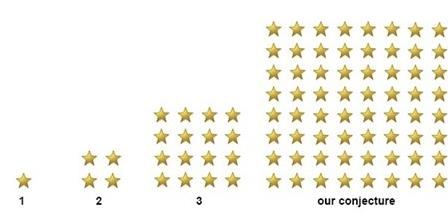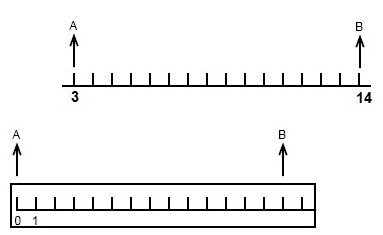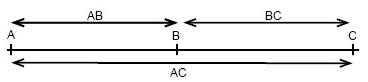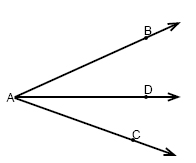Courses

# Proof - General Geometry Mathematics Notes | EduRev

## Mathematics : Proof - General Geometry Mathematics Notes | EduRev

The document Proof - General Geometry Mathematics Notes | EduRev is a part of the Mathematics Course Additional Topics for IIT JAM Mathematics.
All you need of Mathematics at this link: Mathematics

Conjecture
If we look at data over the precipitation in a city for 29 out of 30 days and see that it has been raining every single day it would be a good guess that it will be raining the 30th day as well. A conjecture is an educated guess that is based on known information.
Example
If we are given information about the quantity and formation of section 1, 2 and 3 of stars our conjecture would be as follows.This method to use a number of examples to arrive at a plausible generalization or prediction could also be called inductive reasoning.
If our conjecture would turn out to be false it is called a counterexample.

Working with logic
A true-false statement is any sentence that is either true or false but not both. A negation of a statement has the opposite meaning of a truth value. A negations is written as ~p.
If we call the statement: cucumbers are green, p then:
p: cucumbers are green - this statement is true.
~p: cucumbers are not green - this statement is false.
If we join two statements we can form a compound statement or a conjunction. A conjunction could contain the two statements q and p:
p: cucumbers are green.
q: cucumbers are vegetables.
Conjunctions are noted:
p ∧ q
This is read - p and q. Cucumbers are green and vegetables.
A conjunction is true only if both statements that form the conjunction is true.
If we have two statements that are joined by "or" we have a disjunction.
Example
p: Bill is travelling to Mexico
q: Bill is travelling to Canada
p or q gives us that Bill is travelling to Mexico or Bill is travelling to Canada.
Disjunctions are noted:
p ∨ q
This is read - p or q.
A disjunction is true if at least one of the statements that form it is true.

If-then statement
When we previously discussed inductive reasoning we based our reasoning on examples and on data from earlier events. If we instead use facts, rules and definitions then it's called deductive reasoning.
We will explain this by using an example.
If you get good grades then you will get into a good college.
The part after the "if": you get good grades - is called a hypotheses and the part after the "then" - you will get into a good college - is called a conclusion.
Hypotheses followed by a conclusion is called an If-then statement or a conditional statement.
This is noted as
p → q
This is read - if p then q.
A conditional statement is false if hypothesis is true and the conclusion is false. The example above would be false if it said "if you get good grades then you will not get into a good college".
If we re-arrange a conditional statement or change parts of it then we have what is called a related conditional.

Example
Our conditional statement is: if a population consists of 50% men then 50% of the population must be women.
p → q
If we exchange the position of the hypothesis and the conclusion we get a converse statement: if a population consists of 50% women then 50% of the population must be men.
q → p
If both statements are true or if both statements are false then the converse is true. A conditional and its converse do not mean the same thing
If we negate both the hypothesis and the conclusion we get a inverse statement: if a population do not consist of 50% men then the population do not consist of 50% women.
∼ p → ∼ q
The inverse is not true juest because the conditional is true. The inverse always has the same truth value as the converse.
We could also negate a converse statement, this is called a contrapositive statement:  if a population do not consist of 50% women then the population do not consist of 50% men.
∼ q → ∼ p
The contrapositive does always have the same truth value as the conditional. If the conditional is true then the contrapositive is true.
A pattern of reaoning is a true assumption if it always lead to a true conclusion. The most common patterns of reasoning are detachment and syllogism.
Example
If we turn of the water in the shower, then the water will stop pouring.
If we call the first part p and the second part q then we know that p results in q. This means that if p is true then q will also be true. This is called the law of detachment and is noted:
[(p → q) ∧ p] → q
The law of syllogism tells us that if p → q and q → r then p → r is also true.
This is noted:
[(p → q) ∧ (q → r)] → (p → r)
Example
If the following statements are true:
If we turn of the water (p), then the water will stop pouring (q). If the water stops pouring (q) then we don't get wet any more (r).
Then the law of syllogism tells us that if we turn of the water (p) then we don't get wet (r) must be true.

Proofs using algebra
A two column proof is a method to prove statements using properties that justify each step. The properties are called reasons. All reasons used have been showed in previously algebra courses. We will in the following video lesson show how to prove that x = -½ using the two column proof method.
The ruler postulate tells us that two points on a line can be paired with real numbers so that, given any two points A and B, A is zero and B is a positive real number.Further on the segment addition postulate tells us that if a point B is somewhere between point A and point C then AB + BC = AC.similar relationship is the angle addition postulate. If we have the following angls:then m∠BAD + m∠DAC = m∠BAC

Offer running on EduRev: Apply code STAYHOME200 to get INR 200 off on our premium plan EduRev Infinity!

40 docs

,

,

,

,

,

,

,

,

,

,

,

,

,

,

,

,

,

,

,

,

,

;